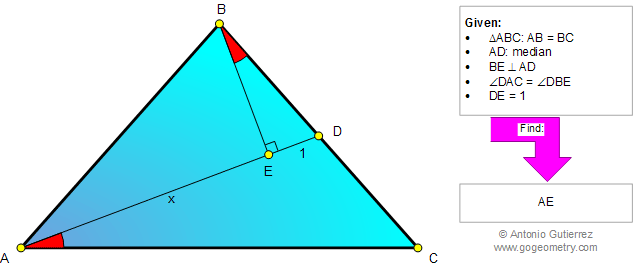###### Online Geometry Problem 867: Isosceles Triangle, Median, Perpendicular, Angle, Congruence. Level: High School, College, Mathematics Education
 The figure below shows a triangle ABC with AB = BC and BE perpendicular to median AD. If angle DAC = angle DBE, and DE = 1, find AE.Home | Search | Geometry | Problems | All Problems | Open Problems | Visual Index | 10 Problems | 861-870 | Triangles | Isosceles Triangle | Median | Perpendicular lines | Angles | Congruence | Email | Solution / comment | By Antonio Gutierrez